Arithmetic
Arithmetic Progression
Associative Property
Averages
Brackets
Closure Property
Commutative Property
Conversion of Measurement Units
Cube Root
Decimal
Divisibility Principles
Equality
Exponents
Factors
Fractions
Fundamental Operations
H.C.F / G.C.D
Integers
L.C.M
Multiples
Multiplicative Identity
Multiplicative Inverse
Numbers
Percentages
Profit and Loss
Ratio and Proportion
Simple Interest
Square Root
Unitary Method
Algebra
Cartesian System
Order Relation
Polynomials
Probability
Standard Identities & their applications
Transpose
Geometry
Basic Geometrical Terms
Circle
Curves
Angles
Define Line, Line Segment and Rays
Non-Collinear Points
Parallelogram
Rectangle
Rhombus
Square
Three dimensional object
Trapezium
Triangle
Trigonometry
Trigonometry Ratios
Data-Handling
Arithmetic Mean
Frequency Distribution Table
Graphs
Median
Mode
Range

Videos
Solved Problems
Home >> Basic Geometrical Terms >> Congruent & Congruence >>

## Define Congruent & Congruence

 Polygons and its Types Sides Vertex Diagonals Adjacent Sides Adjacent Vertices's Perimeter Congruent & Congruence Axis Perpendicular Lines

 Congruent word means "coinciding over each other when superimposed" Congruent object are exact copies of each other. Congruent objects are represented with symbol: ≅ Congruence : The relation of two objects being congruent is called Congruence In the below figure you can see that two cards Ace of Hearts and Ace of Spades are congruent i.e. when both are placed one over other, they coincide exactly.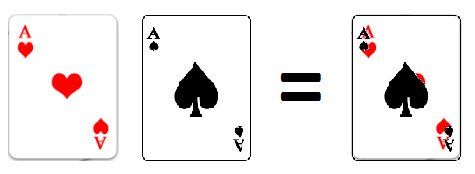In the below figure you can see that two stamps are again congruent to each i.e. when both are placed one over other, they coincide exactly.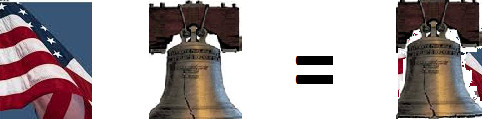Look at the following few more congruent objects: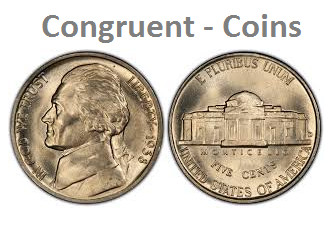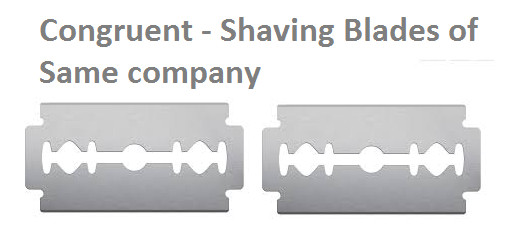Let's study some more examples on congruence Example 1: Observe the following diagram which have two quadrilaterals ABCD and PQRS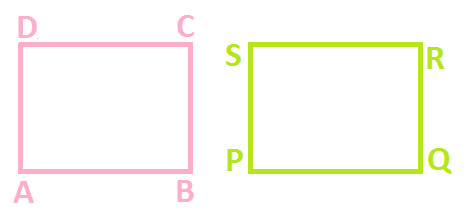Let's superpose PQRS on ABCD (as shown below):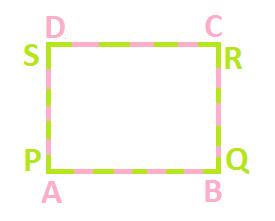You can observe the both the quadrilateral when superpose over one another, covers each other completely. (You can try this activity with the help of tracing paper too) Hence, we can say that Quadrilateral ABCD and PQRS are congruent. Example 2: Observe the following diagram which have two quadrilaterals ABCD and PQRS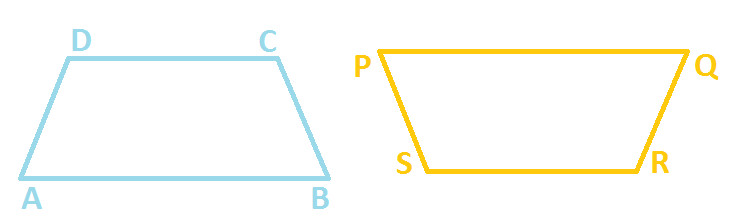Let's superpose PQRS on ABCD (as shown below): Hint: Before superpose you have to flip quadrilateral PQRS vertically.You can observe the both the quadrilateral when superpose over one another, they covers each other completely. (You can try this activity with the help of tracing paper too) Example 3: Observe the following diagram which have two quadrilaterals ABCD and PQRS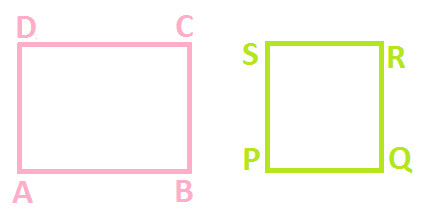Let's superpose PQRS on ABCD (as shown below):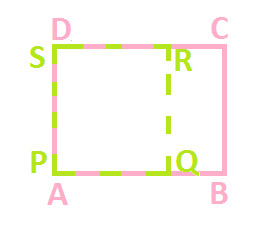You can observe the both the quadrilateral when superpose over one another, does not cover each other completely. (You can try this activity with the help of tracing paper too) Hence, we can say that Quadrilateral ABCD and PQRS are not congruent. Now, in example 1 and 2; figures are congruent because when you can superpose one over another, they cover each other completely. And in example 3, figures are not congruent because when you can superpose one over another, they do not cover each other completely. This also gives us a fact that: Areas of Congruent Figures are always equal or we can say that congruent figures have equal areas. But, the converse of above fact is not true i.e.: Two figures having same areas are not always congruent Example 4: Observe the following figures, Quadrilateral ABCD which is a rectangle and Quadrilateral PQRS which is a square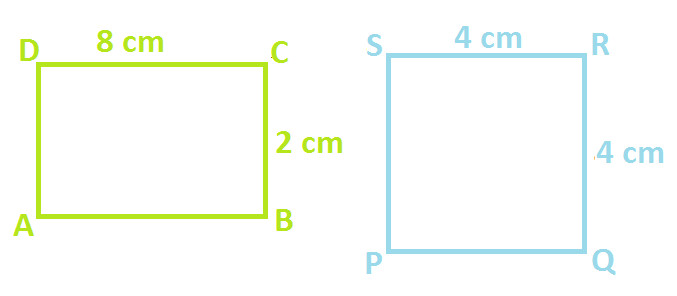Area of rectangle ABCD = 8 X 2 = 16 cm2 Area of square PQRS = 4 X 4 = 16 cm2 You can notice that areas of both the quadrilateral are equal i.e. 16 cm2. But we cannot say that they are congruent. In order to check this, let's superpose one over another as shown below: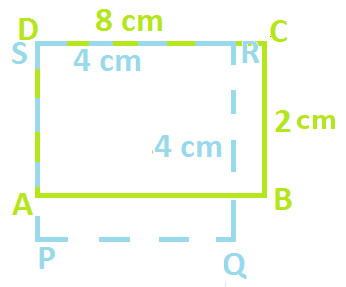You can observe that both the figures when superpose over one another, does not cover each other completely. (You can try this activity with the help of tracing paper too) Hence, this proves the statement that two figures having same areas are not always congruent.

Copyright@2022 Algebraden.com (Math, Algebra & Geometry tutorials for school and home education)